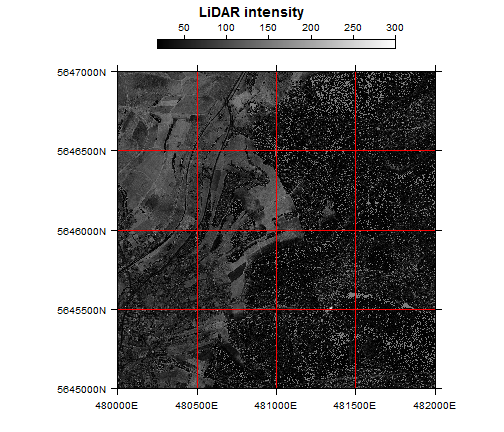BIS-Fogo

Site Tools

en:learning:schools:s01:code-examples:ba-ce-10-01

C10-1 Visualization of spatial data sets in R

The following examples illustrate the visualization of spatial data sets using the sp and raster package.

As always, we start with importing the packages and setting the working directory.

library(sp)
library(raster)
library(RColorBrewer)  # Necessary for individual color maps
library(grid)  # Necessary for plotting the raster grids
## rgdal: version: 0.8-14, (SVN revision 496)
## Geospatial Data Abstraction Library extensions to R successfully loaded
## Loaded GDAL runtime: GDAL 1.10.1, released 2013/08/26
## Path to GDAL shared files: C:/Program Files/R/R-2.15.2/library/rgdal/gdal
## GDAL does not use iconv for recoding strings.
## Loaded PROJ.4 runtime: Rel. 4.8.0, 6 March 2012, [PJ_VERSION: 480]
## Path to PROJ.4 shared files: C:/Program Files/R/R-2.15.2/library/rgdal/proj

setwd("D:/active/moc/dm/programs/r-dm-ln-12")

Visualization of point vectors using sp

A LiDAR data set is supplied as a simple xyz text file. Preprocessing of the data follows the previous examples.

coordinates(lidar) <- ~X + Y
projection(lidar) <- "+proj=utm +zone=32 +ellps=GRS80 +units=m +north"

The Lidar data comes with a classification attribute, which hase the following values:

unique(lidar\$Classification)
##  13  2

Now one can plot the entire data set as maps by just using the spplot command.

spplot(lidar)
## Warning: non-vector columns will be ignored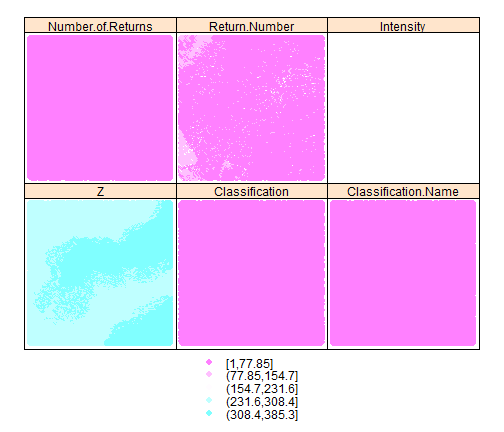To plot only one variable, one has to supply it via the zcol parameter.

spplot(lidar, zcol = "Z")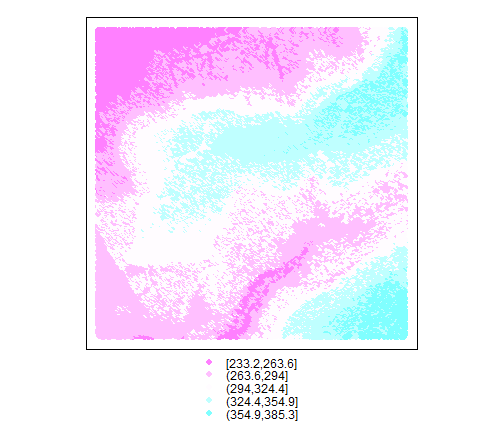As always, the plots are highly configurable. For the example, we define a color pallet first. The next lines define the labels of the map border (using UTM coordinates).

clrs <- colorRampPalette(c(rev(brewer.pal(11, "Spectral")), "black"))

yat = seq(5645000, 5647000, 500)
ylabs = paste(yat, "N", sep = "")
xat = seq(472000, 484000, 500)
xlabs = ifelse(xat < 0, paste(xat, "E", sep = ""), ifelse(xat > 0, paste(xat,
"E", sep = ""), paste(xat, "", sep = "")))
spplot(lidar, zcol = "Z", col.regions = clrs(1000), scales = list(x = list(at = xat,
labels = xlabs), y = list(at = yat, labels = ylabs)), colorkey = TRUE, key.space = list(x = 0.1,
y = 0.1, corner = c(0, 0)), main = "LiDAR dataset, ground returns")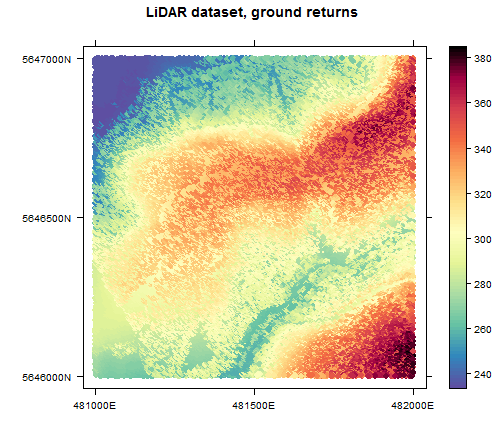Visualization of raster data using raster

From the LiDAR data above we have also derived a raster data set (externally) which gives us the intensity of the LiDAR returns.

Preprocessing is limited to reading the data since it comes with spatial reference information in its meta data. data follows the previous examples.

intensity <- raster("intensity_1rt1.tif", native = T)
projection(lidar)
##  "+proj=utm +zone=32 +ellps=GRS80 +units=m"

The raster package supplies both the plot and spplot function. For a simple plot, the former is all you need (here a user-defined color palette is added).

plot(intensity)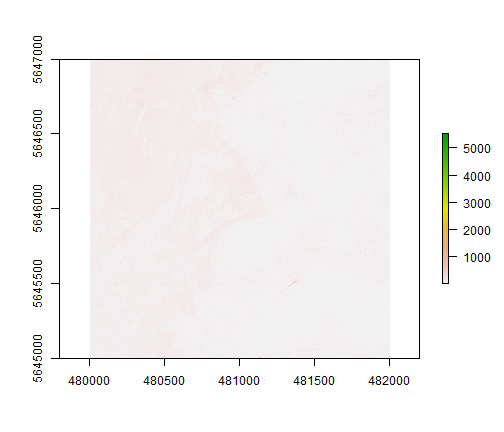Again, more sophisticated plots are possible (with spplot). For the map borders, we use the information from above again. Parameter at defines the range of the data values which are stretchted to the grey values.

intensity.plot <- spplot(intensity, maxpixels = 4e+05, main = "LiDAR intensity",
col.regions = colorRampPalette(c("black", "white")), at = seq(18, 300),
panel = function(...) {
grid.rect(gp = gpar(col = NA, fill = "grey50"))
panel.levelplot(...)
}, scales = list(x = list(at = xat, labels = xlabs), y = list(at = yat,
labels = ylabs)), colorkey = list(space = "top", width = 1, height = 0.75))
print(intensity.plot)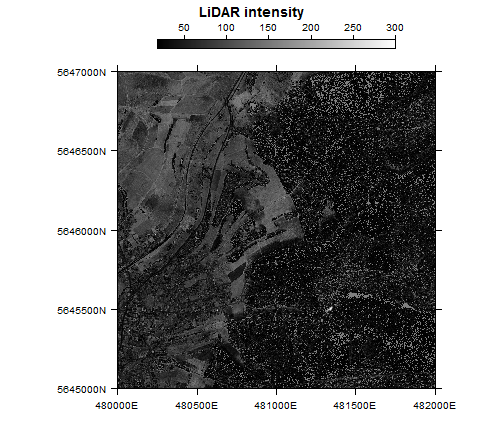Visualization of polygon vector data using sp

The last example shows the visualization of a polygon vector file in ESRI's shape format.

Since this shape file comes with spatial reference, preprocessing is again restricted to reading the data.

polygon <- readOGR("polygon.shp", layer = "polygon")
## OGR data source with driver: ESRI Shapefile
## Source: "polygon.shp", layer: "polygon"
## with 16 features and 3 fields
## Feature type: wkbPolygon with 2 dimensions

For plooting we use spplot again.

polygon.plot <- spplot(polygon[, 1], col.regions = FALSE, col = "red")
print(polygon.plot)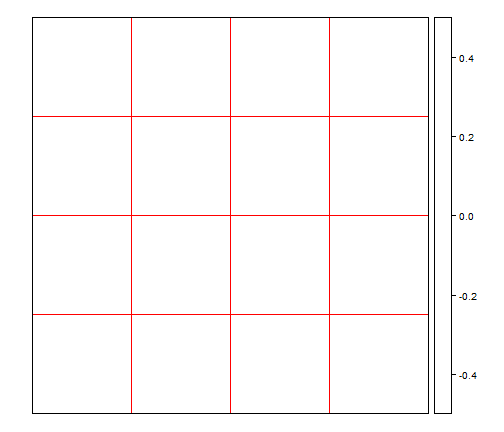As one can see, the polygon just consists of several grid boxes. Let's overlay it to the map of the intensity data from above.

print(intensity.plot + as.layer(polygon.plot))